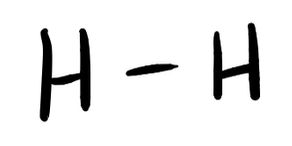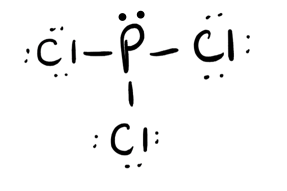# 3.3.1: Lewis Structures (Problems)

$$\newcommand{\vecs}{\overset { \rightharpoonup} {\mathbf{#1}} }$$ $$\newcommand{\vecd}{\overset{-\!-\!\rightharpoonup}{\vphantom{a}\smash {#1}}}$$$$\newcommand{\id}{\mathrm{id}}$$ $$\newcommand{\Span}{\mathrm{span}}$$ $$\newcommand{\kernel}{\mathrm{null}\,}$$ $$\newcommand{\range}{\mathrm{range}\,}$$ $$\newcommand{\RealPart}{\mathrm{Re}}$$ $$\newcommand{\ImaginaryPart}{\mathrm{Im}}$$ $$\newcommand{\Argument}{\mathrm{Arg}}$$ $$\newcommand{\norm}{\| #1 \|}$$ $$\newcommand{\inner}{\langle #1, #2 \rangle}$$ $$\newcommand{\Span}{\mathrm{span}}$$ $$\newcommand{\id}{\mathrm{id}}$$ $$\newcommand{\Span}{\mathrm{span}}$$ $$\newcommand{\kernel}{\mathrm{null}\,}$$ $$\newcommand{\range}{\mathrm{range}\,}$$ $$\newcommand{\RealPart}{\mathrm{Re}}$$ $$\newcommand{\ImaginaryPart}{\mathrm{Im}}$$ $$\newcommand{\Argument}{\mathrm{Arg}}$$ $$\newcommand{\norm}{\| #1 \|}$$ $$\newcommand{\inner}{\langle #1, #2 \rangle}$$ $$\newcommand{\Span}{\mathrm{span}}$$$$\newcommand{\AA}{\unicode[.8,0]{x212B}}$$

PROBLEM $$\PageIndex{1}$$

Write Lewis structures for the following: (please note, none of the solutions are using the expanded octet rule or formal charges)

1. H2
2. HBr
3. PCl3
4. SF2
5. H2CCH2
6. HNNH
7. H2CNH
8. NO
9. N2
10. CO
11. CNPROBLEM $$\PageIndex{2}$$

Write Lewis structures for the following: (please note, none of the solutions are using the expanded octet rule or formal charges)

1. O2
2. H2CO
3. ClNO
4. SiCl4
5. H3O+
6. $$\ce{NH4+}$$
7. $$\ce{BF4-}$$
8. HCCH
9. ClCN
10. $$\ce{C2^2+}$$PROBLEM $$\PageIndex{3}$$

Write Lewis structures for: (please note, none of the solutions are using the expanded octet rule or formal charges)

a. $$\ce{PO4^3-}$$
c. $$\ce{SO3^2-}$$

d. HONOPROBLEM $$\PageIndex{4}$$

Methanol, H3COH, is used as the fuel in some race cars. Ethanol, C2H5OH, is used extensively as motor fuel in Brazil. Both methanol and ethanol produce CO2 and H2O when they burn. Write the chemical equations for these combustion reactions using Lewis structures instead of chemical formulas.PROBLEM $$\PageIndex{5}$$

Many planets in our solar system contain organic chemicals including methane (CH4) and traces of ethylene (C2H4), ethane (C2H6), propyne (H3CCCH), and diacetylene (HCCCCH). Write the Lewis structures for each of these molecules. (diacetylene may be a little tricky!)PROBLEM $$\PageIndex{6}$$

Carbon tetrachloride was formerly used in fire extinguishers for electrical fires. It is no longer used for this purpose because of the formation of the toxic gas phosgene, Cl2CO. Write the Lewis structures for carbon tetrachloride and phosgene.PROBLEM $$\PageIndex{7}$$

The arrangement of atoms in several biologically important molecules is given here. Complete the Lewis structures of these molecules by adding multiple bonds and lone pairs. Do not add any more atoms.

a. the amino acid serine:b. urea:c. pyruvic acid:d. uracil:e. carbonic acid:PROBLEM $$\PageIndex{8}$$

How are single, double, and triple bonds similar? How do they differ?